# COP 4710 Database Systems Spring 2006 Chapter 19

• Slides: 47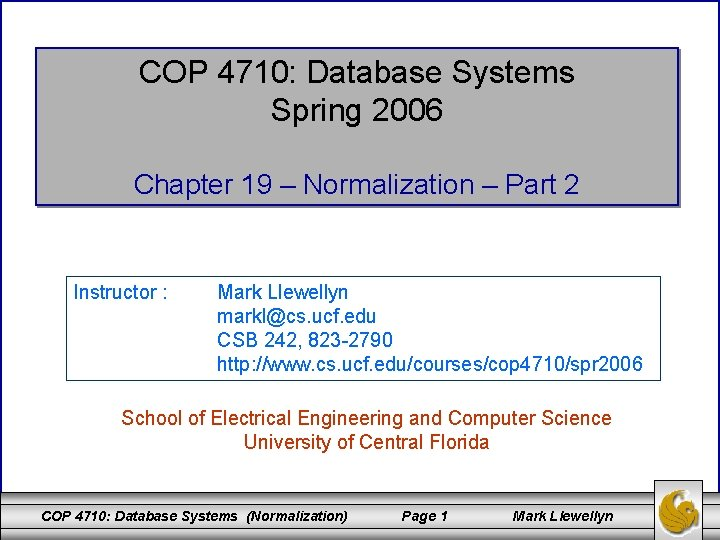COP 4710: Database Systems Spring 2006 Chapter 19 – Normalization – Part 2 Instructor : Mark Llewellyn [email protected] ucf. edu CSB 242, 823 -2790 http: //www. cs. ucf. edu/courses/cop 4710/spr 2006 School of Electrical Engineering and Computer Science University of Central Florida COP 4710: Database Systems (Normalization) Page 1 Mark Llewellyn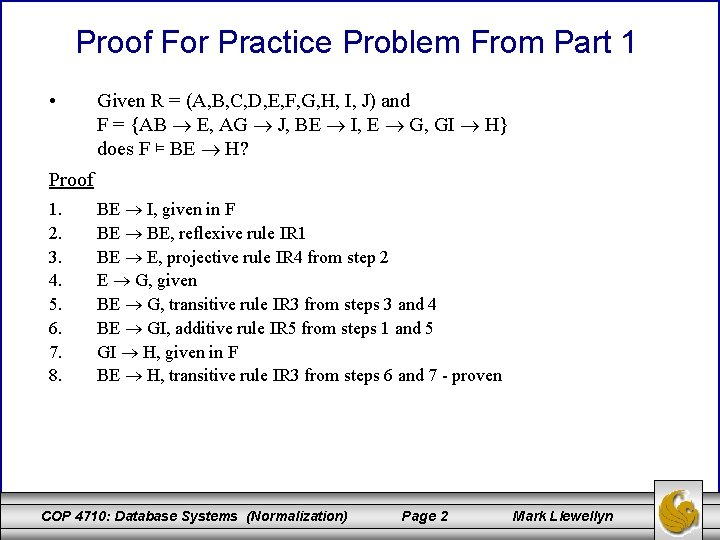Proof For Practice Problem From Part 1 • Given R = (A, B, C, D, E, F, G, H, I, J) and F = {AB E, AG J, BE I, E G, GI H} does F ⊨ BE H? Proof 1. 2. 3. 4. 5. 6. 7. 8. BE I, given in F BE BE, reflexive rule IR 1 BE E, projective rule IR 4 from step 2 E G, given BE G, transitive rule IR 3 from steps 3 and 4 BE GI, additive rule IR 5 from steps 1 and 5 GI H, given in F BE H, transitive rule IR 3 from steps 6 and 7 - proven COP 4710: Database Systems (Normalization) Page 2 Mark LlewellynDetermining the Keys of a Relation Schema • • If R is a relational schema with attributes A 1, A 2, . . . , An and a set of functional dependencies F where X ⊆ {A 1, A 2, . . . , An} then X is a key of R if: 1. X → A 1 A 2. . . An F+, and 2. no proper subset Y ⊆ X gives Y → A 1 A 2. . . An F+. Basically, this definition means that you must attempt to generate the closure of all possible subsets of the schema of R and determine which sets produce all of the attributes in the schema. COP 4710: Database Systems (Normalization) Page 3 Mark LlewellynDetermining Keys - Example Let r = (C, T, H, R, S, G) with F = {C T, HR C, HT R, CS G, HS R} Step 1: Generate (Ai)+ for 1 i n C+ = {CT}, T+ = {T}, H+ = {H} R+ = {R}, S+ = {S}, G+ = {G} no single attribute is a key for R Step 2: Generate (Ai. Aj)+ for 1 i n, 1 j n (CT)+ = {C, T}, (CH)+ = {CHTR}, (CR)+ = {CRT} (CS)+ = {CSGT}, (CG)+ = {CGT}, (TH)+ = {THRC} (TR)+ = {TR}, (TS)+ = {TS}, (TG)+ = {TG} (HR)+ = {HRCT}, (HS)+ = {HSRCTG}, (HG)+ = {HG} (RS)+ = {RS}, (RG)+ = {RG}, (SG)+ = {SG} The attribute set (HS) is a key for R COP 4710: Database Systems (Normalization) Page 4 Mark LlewellynDetermining Keys - Example Step 3: Generate (Ai. Aj. Ak)+ for 1 i n, 1 j n, 1 k n (CTH)+ = {CTHR}, (CTR)+ = {CTR} (CTS)+ = {CTSG}, (CTG)+ = {CTG} (CHR)+ = {CHRT}, (CHS)+ = {CHSTRG} (CHG)+ = {CHGTR}, (CRS)+ = {CRSTG} (CRG)+ = {CRGT}, (CSG)+ = {CSGT} (THR)+ = {THRC}, (THS)+ = {THSRCG} (THG)+ = {THGRC}, (TRS)+ = {TRS} (TRG)+ = {TRG}, (TSG)+ = {TSG} (HRS)+ = {HRSCTG}, (HRG)+ = {HRGCT} (HSG)+ = {HSGRCT}, (RSG)+ = {RSG} Superkeys are shown in red. COP 4710: Database Systems (Normalization) Page 5 Mark Llewellyn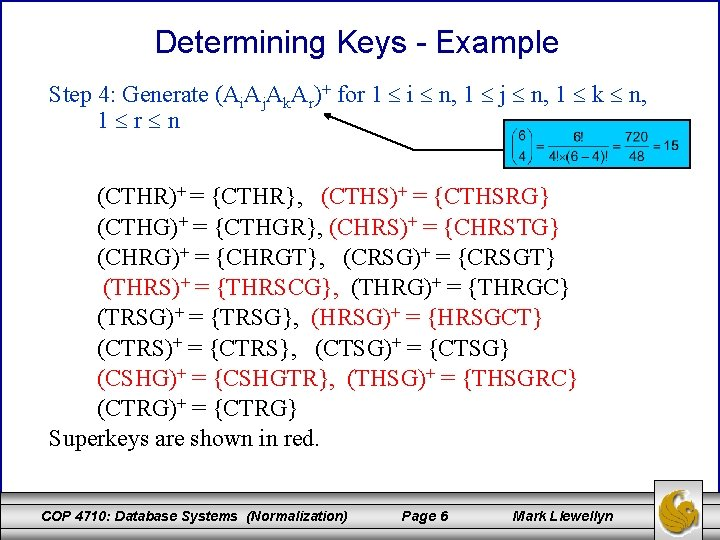Determining Keys - Example Step 4: Generate (Ai. Aj. Ak. Ar)+ for 1 i n, 1 j n, 1 k n, 1 r n (CTHR)+ = {CTHR}, (CTHS)+ = {CTHSRG} (CTHG)+ = {CTHGR}, (CHRS)+ = {CHRSTG} (CHRG)+ = {CHRGT}, (CRSG)+ = {CRSGT} (THRS)+ = {THRSCG}, (THRG)+ = {THRGC} (TRSG)+ = {TRSG}, (HRSG)+ = {HRSGCT} (CTRS)+ = {CTRS}, (CTSG)+ = {CTSG} (CSHG)+ = {CSHGTR}, (THSG)+ = {THSGRC} (CTRG)+ = {CTRG} Superkeys are shown in red. COP 4710: Database Systems (Normalization) Page 6 Mark LlewellynDetermining Keys - Example Step 5: Generate (Ai. Aj. Ak. Ar. As)+ for 1 i n, 1 j n, 1 k n, 1 r n, 1 s n (CTHRS)+ = {CTHSRG} (CTHRG)+ = {CTHGR} (CTHSG)+ = {CTHSGR} (CHRSG)+ = {CHRSGT} (CTRSG)+ = {CTRSG} (THRSG)+ = {THRSGC} Superkeys are shown in red. COP 4710: Database Systems (Normalization) Page 7 Mark Llewellyn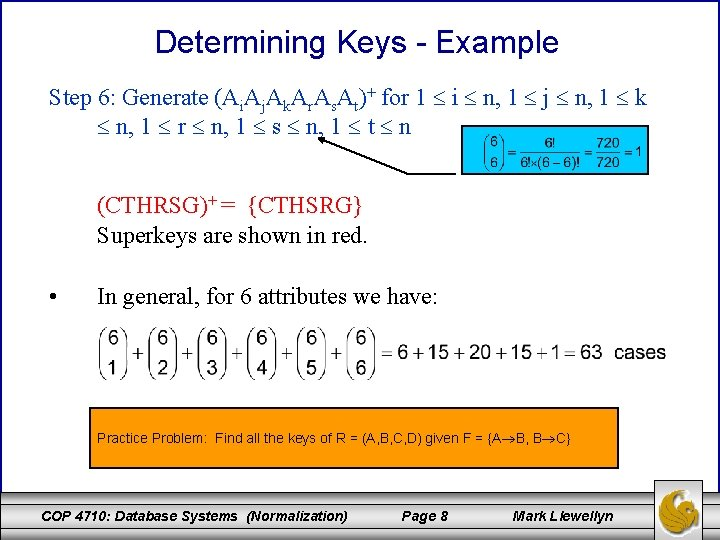Determining Keys - Example Step 6: Generate (Ai. Aj. Ak. Ar. As. At)+ for 1 i n, 1 j n, 1 k n, 1 r n, 1 s n, 1 t n (CTHRSG)+ = {CTHSRG} Superkeys are shown in red. • In general, for 6 attributes we have: Practice Problem: Find all the keys of R = (A, B, C, D) given F = {A B, B C} COP 4710: Database Systems (Normalization) Page 8 Mark Llewellyn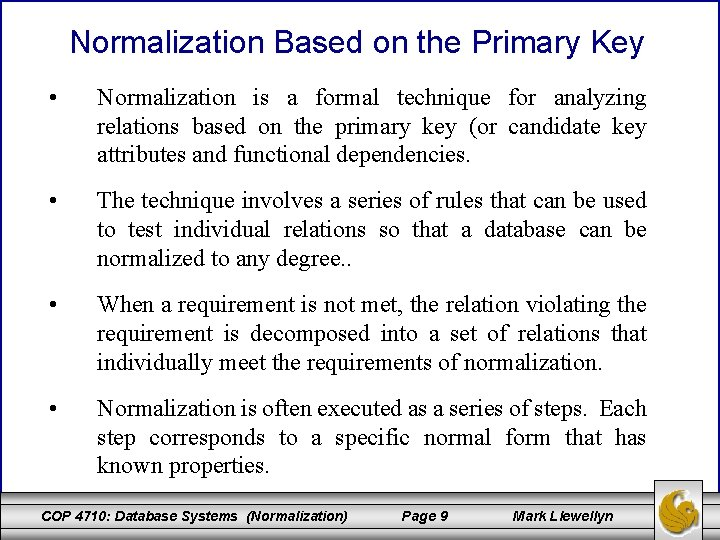Normalization Based on the Primary Key • Normalization is a formal technique for analyzing relations based on the primary key (or candidate key attributes and functional dependencies. • The technique involves a series of rules that can be used to test individual relations so that a database can be normalized to any degree. . • When a requirement is not met, the relation violating the requirement is decomposed into a set of relations that individually meet the requirements of normalization. • Normalization is often executed as a series of steps. Each step corresponds to a specific normal form that has known properties. COP 4710: Database Systems (Normalization) Page 9 Mark LlewellynRelationship Between Normal Forms N 1 NF 2 NF 3 NF BCNF 4 NF 5 NF Higher Normal Forms COP 4710: Database Systems (Normalization) Page 10 Mark Llewellyn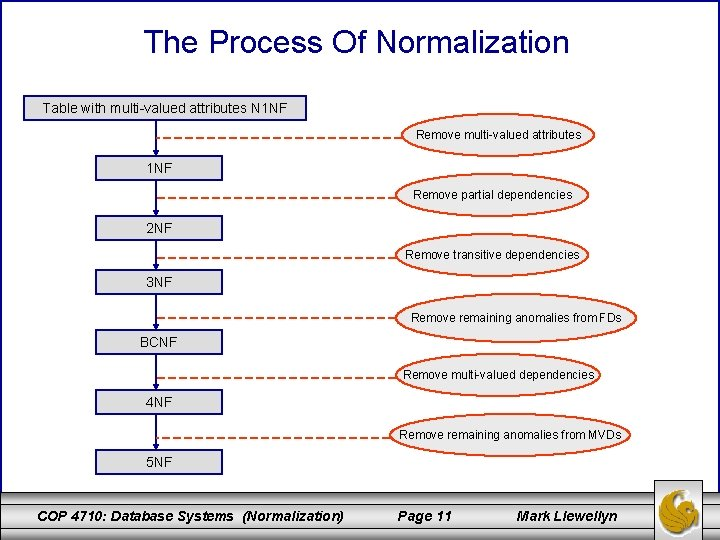The Process Of Normalization Table with multi-valued attributes N 1 NF Remove multi-valued attributes 1 NF Remove partial dependencies 2 NF Remove transitive dependencies 3 NF Remove remaining anomalies from FDs BCNF Remove multi-valued dependencies 4 NF Remove remaining anomalies from MVDs 5 NF COP 4710: Database Systems (Normalization) Page 11 Mark LlewellynNormalization Requirements • For the relational model it is important to recognize that it is only first normal form (1 NF) that is critical in creating relations. All the subsequent normal forms are optional. • However, to avoid the update anomalies that we discussed earlier, it is normally recommended that the database designer proceed to at least 3 NF. • As the figure on the previous page illustrates, some 1 NF relations are also in 2 NF and some 2 NF relations are also in 3 NF, and so on. • As we proceed, we’ll look at the requirements for each normal form and a decomposition technique to achieve relation schemas in that normal form. COP 4710: Database Systems (Normalization) Page 12 Mark LlewellynNon-First Normal Form (N 1 NF) • Non-first normal form relation are those relations in which one or more of the attributes are non-atomic. In other words, within a relation and within a single tuple there is a multi-valued attribute. • There are several important extensions to the relational model in which N 1 NF relations are utilized. For the most part these go beyond the scope of this course and we will not discuss them in any significant detail. Temporal relational databases and certain categories of spatial databases fall into the N 1 NF category. COP 4710: Database Systems (Normalization) Page 13 Mark Llewellyn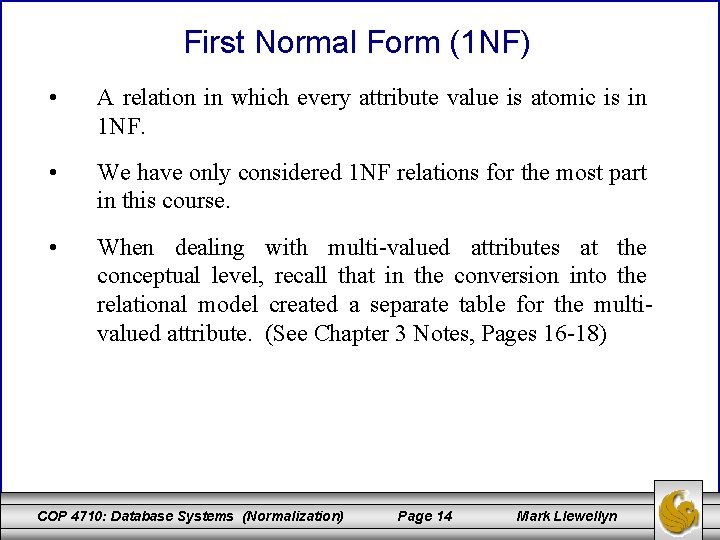First Normal Form (1 NF) • A relation in which every attribute value is atomic is in 1 NF. • We have only considered 1 NF relations for the most part in this course. • When dealing with multi-valued attributes at the conceptual level, recall that in the conversion into the relational model created a separate table for the multivalued attribute. (See Chapter 3 Notes, Pages 16 -18) COP 4710: Database Systems (Normalization) Page 14 Mark Llewellyn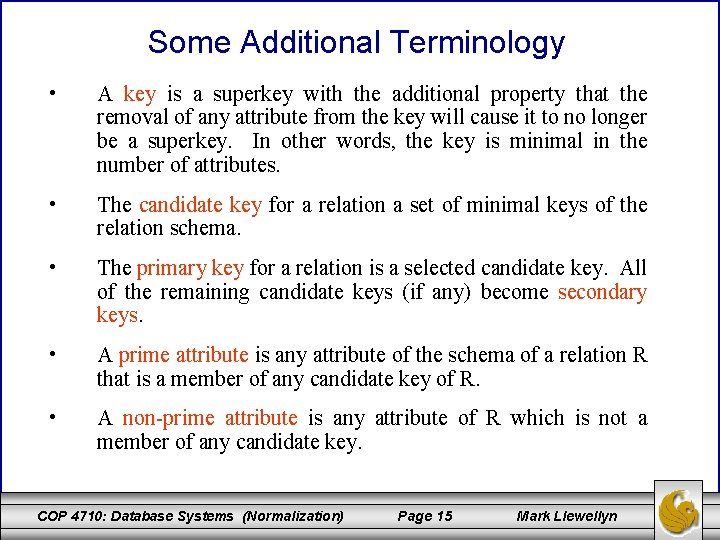Some Additional Terminology • A key is a superkey with the additional property that the removal of any attribute from the key will cause it to no longer be a superkey. In other words, the key is minimal in the number of attributes. • The candidate key for a relation a set of minimal keys of the relation schema. • The primary key for a relation is a selected candidate key. All of the remaining candidate keys (if any) become secondary keys. • A prime attribute is any attribute of the schema of a relation R that is a member of any candidate key of R. • A non-prime attribute is any attribute of R which is not a member of any candidate key. COP 4710: Database Systems (Normalization) Page 15 Mark Llewellyn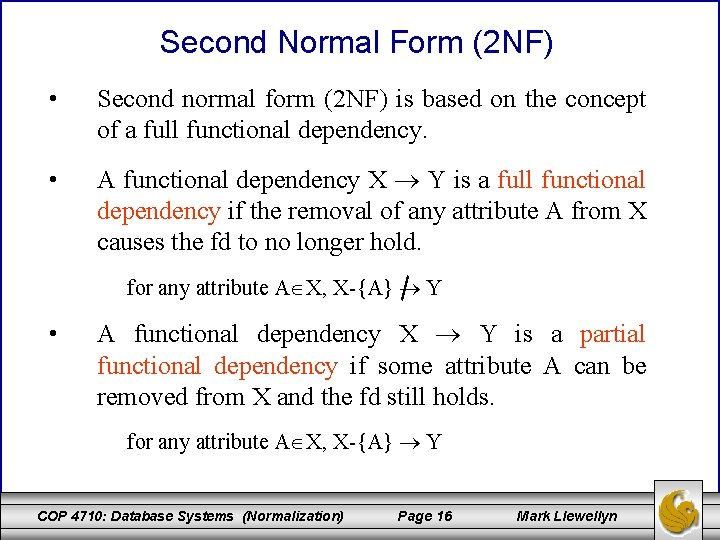Second Normal Form (2 NF) • Second normal form (2 NF) is based on the concept of a full functional dependency. • A functional dependency X Y is a full functional dependency if the removal of any attribute A from X causes the fd to no longer hold. for any attribute A X, X-{A} Y • A functional dependency X Y is a partial functional dependency if some attribute A can be removed from X and the fd still holds. for any attribute A X, X-{A} Y COP 4710: Database Systems (Normalization) Page 16 Mark LlewellynDefinition of Second Normal Form (2 NF) • A relation scheme R is in 2 NF with respect to a set of functional dependencies F if every non-prime attribute is fully dependent on every key of R. • Another way of stating this is: there does not exist a non-prime attribute which is partially dependent on any key of R. In other words, no non-prime attribute is dependent on only a portion of the key of R. COP 4710: Database Systems (Normalization) Page 17 Mark Llewellyn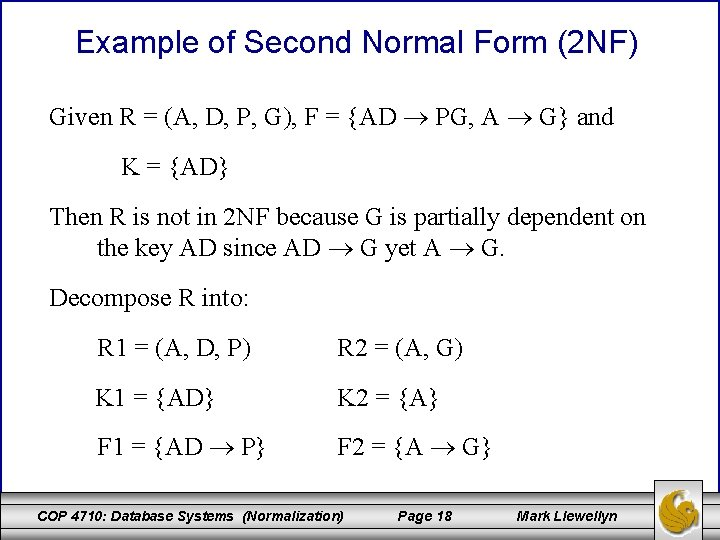Example of Second Normal Form (2 NF) Given R = (A, D, P, G), F = {AD PG, A G} and K = {AD} Then R is not in 2 NF because G is partially dependent on the key AD since AD G yet A G. Decompose R into: R 1 = (A, D, P) R 2 = (A, G) K 1 = {AD} K 2 = {A} F 1 = {AD P} F 2 = {A G} COP 4710: Database Systems (Normalization) Page 18 Mark Llewellyn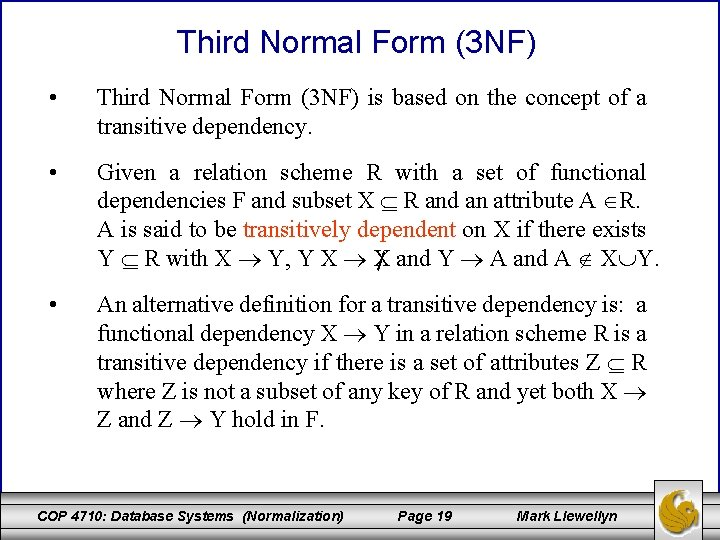Third Normal Form (3 NF) • Third Normal Form (3 NF) is based on the concept of a transitive dependency. • Given a relation scheme R with a set of functional dependencies F and subset X R and an attribute A R. A is said to be transitively dependent on X if there exists Y R with X Y, Y X X and Y A and A X Y. • An alternative definition for a transitive dependency is: a functional dependency X Y in a relation scheme R is a transitive dependency if there is a set of attributes Z R where Z is not a subset of any key of R and yet both X Z and Z Y hold in F. COP 4710: Database Systems (Normalization) Page 19 Mark Llewellyn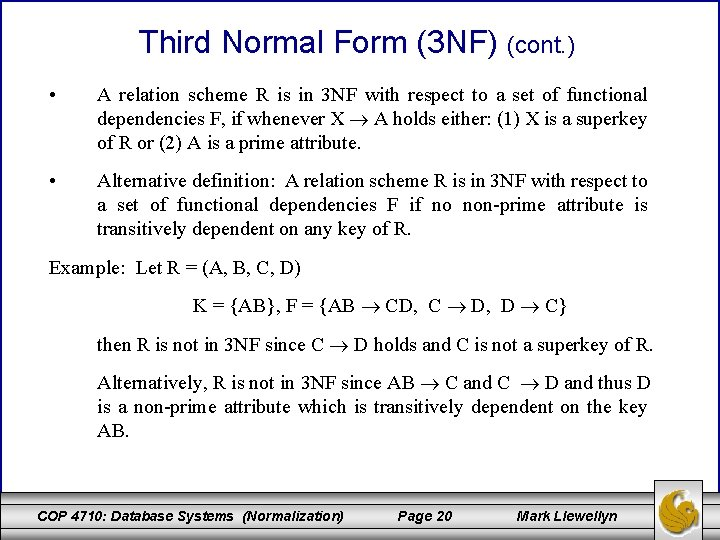Third Normal Form (3 NF) (cont. ) • A relation scheme R is in 3 NF with respect to a set of functional dependencies F, if whenever X A holds either: (1) X is a superkey of R or (2) A is a prime attribute. • Alternative definition: A relation scheme R is in 3 NF with respect to a set of functional dependencies F if no non-prime attribute is transitively dependent on any key of R. Example: Let R = (A, B, C, D) K = {AB}, F = {AB CD, C D, D C} then R is not in 3 NF since C D holds and C is not a superkey of R. Alternatively, R is not in 3 NF since AB C and C D and thus D is a non-prime attribute which is transitively dependent on the key AB. COP 4710: Database Systems (Normalization) Page 20 Mark LlewellynWhy Third Normal Form? • What does 3 NF do for us? database: Consider the following assign(flight, day, pilot-id, pilot-name) K = {flight day} F = {pilot-id pilot-name, pilot-name pilot-id} flight day pilot-id pilot-name 112 Feb. 11 317 Mark 112 Feb. 12 246 Kristi 114 Feb. 13 317 Mark COP 4710: Database Systems (Normalization) Page 21 Mark Llewellyn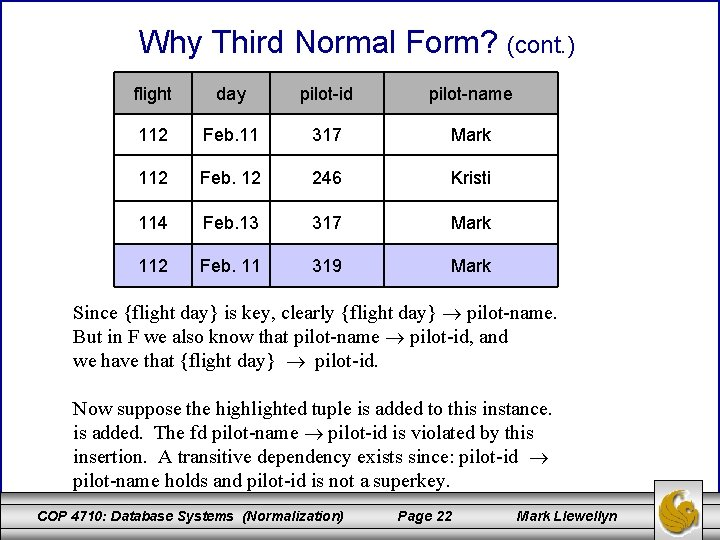Why Third Normal Form? (cont. ) flight day pilot-id pilot-name 112 Feb. 11 317 Mark 112 Feb. 12 246 Kristi 114 Feb. 13 317 Mark 112 Feb. 11 319 Mark Since {flight day} is key, clearly {flight day} pilot-name. But in F we also know that pilot-name pilot-id, and we have that {flight day} pilot-id. Now suppose the highlighted tuple is added to this instance. is added. The fd pilot-name pilot-id is violated by this insertion. A transitive dependency exists since: pilot-id pilot-name holds and pilot-id is not a superkey. COP 4710: Database Systems (Normalization) Page 22 Mark Llewellyn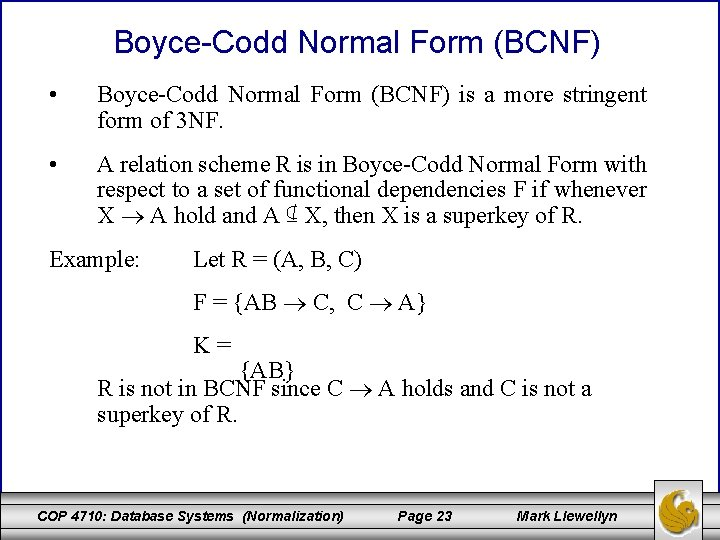Boyce-Codd Normal Form (BCNF) • Boyce-Codd Normal Form (BCNF) is a more stringent form of 3 NF. • A relation scheme R is in Boyce-Codd Normal Form with respect to a set of functional dependencies F if whenever X A hold and A ⊈ X, then X is a superkey of R. Example: Let R = (A, B, C) F = {AB C, C A} K= {AB} R is not in BCNF since C A holds and C is not a superkey of R. COP 4710: Database Systems (Normalization) Page 23 Mark Llewellyn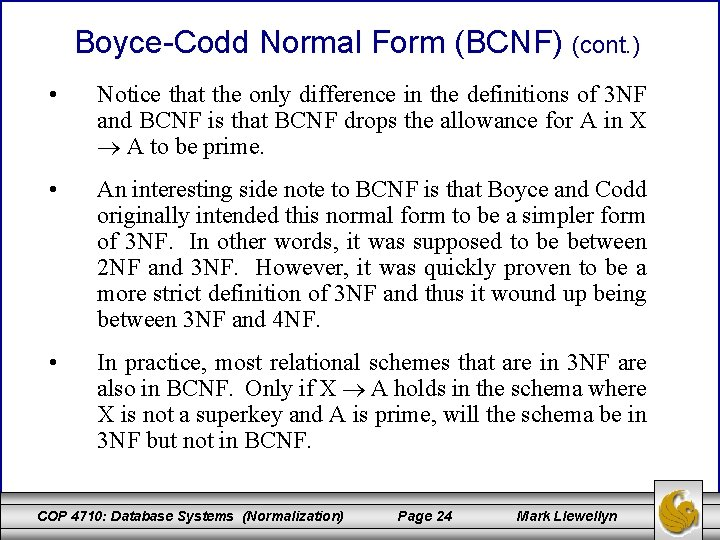Boyce-Codd Normal Form (BCNF) (cont. ) • Notice that the only difference in the definitions of 3 NF and BCNF is that BCNF drops the allowance for A in X A to be prime. • An interesting side note to BCNF is that Boyce and Codd originally intended this normal form to be a simpler form of 3 NF. In other words, it was supposed to be between 2 NF and 3 NF. However, it was quickly proven to be a more strict definition of 3 NF and thus it wound up being between 3 NF and 4 NF. • In practice, most relational schemes that are in 3 NF are also in BCNF. Only if X A holds in the schema where X is not a superkey and A is prime, will the schema be in 3 NF but not in BCNF. COP 4710: Database Systems (Normalization) Page 24 Mark Llewellyn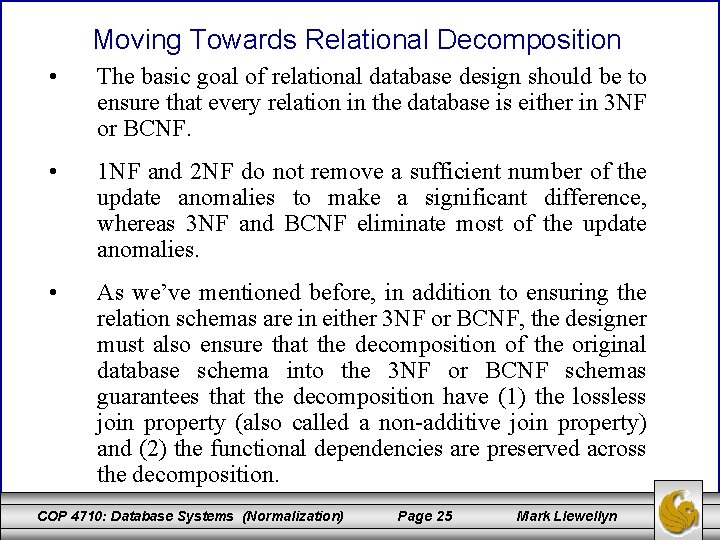Moving Towards Relational Decomposition • The basic goal of relational database design should be to ensure that every relation in the database is either in 3 NF or BCNF. • 1 NF and 2 NF do not remove a sufficient number of the update anomalies to make a significant difference, whereas 3 NF and BCNF eliminate most of the update anomalies. • As we’ve mentioned before, in addition to ensuring the relation schemas are in either 3 NF or BCNF, the designer must also ensure that the decomposition of the original database schema into the 3 NF or BCNF schemas guarantees that the decomposition have (1) the lossless join property (also called a non-additive join property) and (2) the functional dependencies are preserved across the decomposition. COP 4710: Database Systems (Normalization) Page 25 Mark LlewellynMoving Towards Relational Decomposition (cont. ) • There are decomposition algorithms that will guarantee a 3 NF decomposition which ensures both the lossless join property and preservation of the functional dependencies. • However, there is no algorithm which will guarantee a BCNF decomposition which ensures both the lossless join property and preserves the functional dependencies. There is an algorithm that will guarantee BCNF and the lossless join property, but this algorithm cannot guarantee that the dependencies will be preserved. • It is for this reason that many times, 3 NF is as strong a normal form as will be possible for a certain set of schemas, since an attempt to force BCNF may result in the nonpreservation of the dependencies. • In the next few pages we’ll look at these two properties more closely. COP 4710: Database Systems (Normalization) Page 26 Mark Llewellyn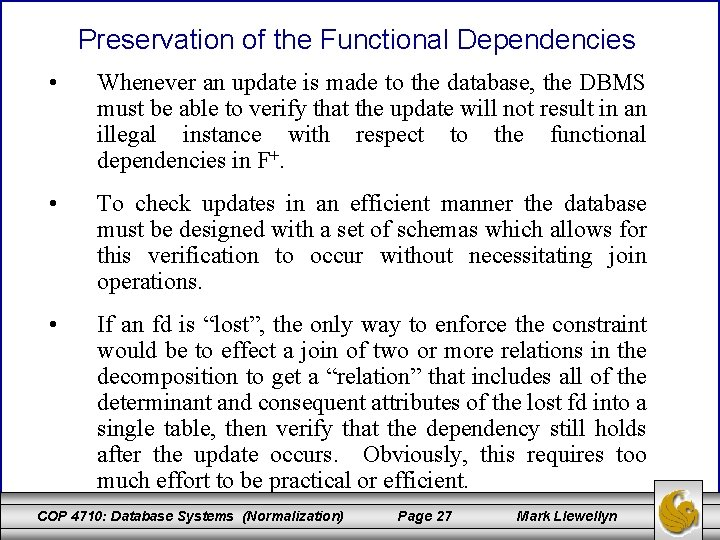Preservation of the Functional Dependencies • Whenever an update is made to the database, the DBMS must be able to verify that the update will not result in an illegal instance with respect to the functional dependencies in F+. • To check updates in an efficient manner the database must be designed with a set of schemas which allows for this verification to occur without necessitating join operations. • If an fd is “lost”, the only way to enforce the constraint would be to effect a join of two or more relations in the decomposition to get a “relation” that includes all of the determinant and consequent attributes of the lost fd into a single table, then verify that the dependency still holds after the update occurs. Obviously, this requires too much effort to be practical or efficient. COP 4710: Database Systems (Normalization) Page 27 Mark LlewellynPreservation of the Functional Dependencies (cont. ) • Informally, the preservation of the dependencies means that if X Y from F appears either explicitly in one of the relational schemas in the decomposition scheme or can be inferred from the dependencies that appear in some relational schema within the decomposition scheme, then the original set of dependencies would be preserved on the decomposition scheme. • It is important to note that what is required to preserve the dependencies is not that every fd in F be explicitly present in some relation schema in the decomposition, but rather the union of all the dependencies that hold on all of the individual relation schemas in the decomposition be equivalent to F (recall what equivalency means in this context). COP 4710: Database Systems (Normalization) Page 28 Mark Llewellyn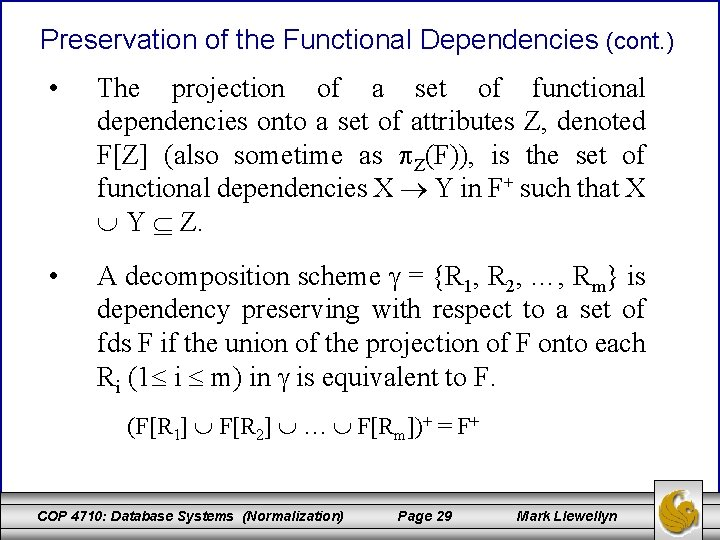Preservation of the Functional Dependencies (cont. ) • The projection of a set of functional dependencies onto a set of attributes Z, denoted F[Z] (also sometime as Z(F)), is the set of functional dependencies X Y in F+ such that X Y Z. • A decomposition scheme = {R 1, R 2, …, Rm} is dependency preserving with respect to a set of fds F if the union of the projection of F onto each Ri (1 i m) in is equivalent to F. (F[R 1] F[R 2] … F[Rm])+ = F+ COP 4710: Database Systems (Normalization) Page 29 Mark Llewellyn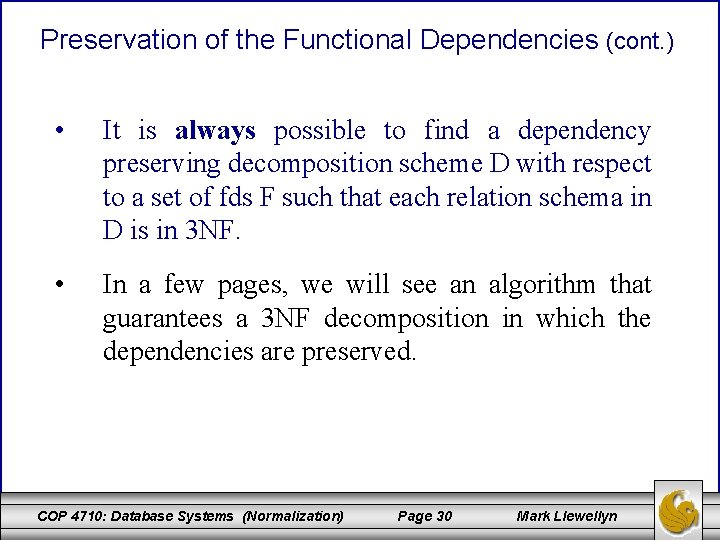Preservation of the Functional Dependencies (cont. ) • It is always possible to find a dependency preserving decomposition scheme D with respect to a set of fds F such that each relation schema in D is in 3 NF. • In a few pages, we will see an algorithm that guarantees a 3 NF decomposition in which the dependencies are preserved. COP 4710: Database Systems (Normalization) Page 30 Mark LlewellynAlgorithm for Testing the Preservation of Dependencies Algorithm Preserve // input: a decomposition D= (R 1, R 2, …, Rk), a set of fds F, an fd X Y // output: true if D preserves F, false otherwise Preserve (D , F, X Y) Z = X; while (changes to Z occur) do for i = 1 to k do // there are k schemas in D Z = Z ( (Z Ri )+ Ri ) endfor; endwhile; if Y Z then return true; // Z ⊨ X Y else return false; end. COP 4710: Database Systems (Normalization) Page 31 Mark LlewellynHow Algorithm Preserves Works • The set Z which is computed is basically the following: • Note that G is not actually computed but merely tested to see if G covers F. To test if G covers F we need to consider each fd X Y in F and determine if contains Y. • Thus, the technique is to compute without having G available by repeatedly considering the effect of closing F with respect to the projections of F onto the various Ri. COP 4710: Database Systems (Normalization) Page 32 Mark LlewellynA Hugmongously Big Example Let R = (A, B, C, D) F = {A B, B C, C D, D A} D = {(AB), (BC), (CD)} G = F[AB] F[BC] F[CD] Z = Z ((Z Ri)+ Ri) Test for each fd in F. Test for A B Z = A, = {A} ((A AB)+ AB) = {A} ((A)+ AB) = {A} (ABCD AB) = {A} {AB} = {AB} COP 4710: Database Systems (Normalization) Page 33 Mark Llewellyn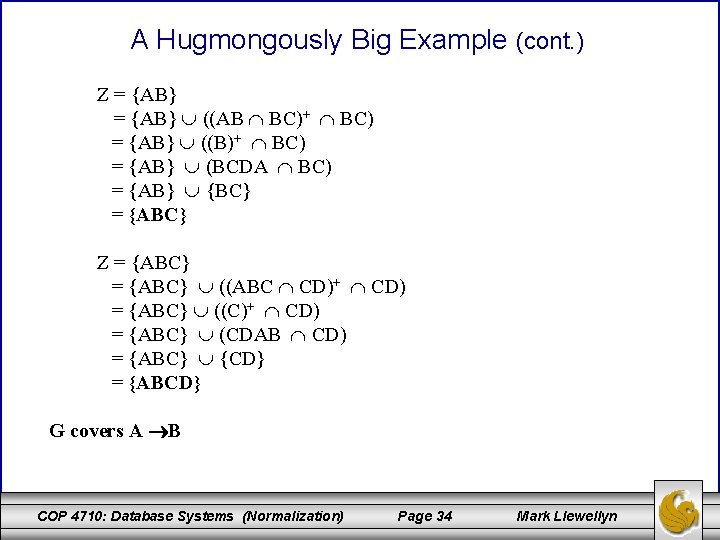A Hugmongously Big Example (cont. ) Z = {AB} ((AB BC)+ BC) = {AB} ((B)+ BC) = {AB} (BCDA BC) = {AB} {BC} = {ABC} Z = {ABC} ((ABC CD)+ CD) = {ABC} ((C)+ CD) = {ABC} (CDAB CD) = {ABC} {CD} = {ABCD} G covers A B COP 4710: Database Systems (Normalization) Page 34 Mark LlewellynA Hugmongously Big Example (cont. ) Test for B C Z = B, = {B} ((B AB)+ AB) = {B} ((B)+ AB) = {B} (BCDA AB) = {B} {AB} = {AB} Z = {AB} ((AB BC)+ BC) = {AB} ((B)+ BC) = {AB} (BCDA BC) = {AB} {BC} = {ABC} Z = {ABC} ((ABC CD)+ CD) = {ABC} ((C)+ CD) = {ABC} (CDAB CD) = {ABC} {CD} = {ABC} So G covers B C COP 4710: Database Systems (Normalization) Page 35 Mark LlewellynA Hugmongously Big Example (cont. ) Test for C D Z = C, = {C} ((C AB)+ AB) = {C} (( )+ AB) = {C} ( ) = {C} Z = {C} ((C BC)+ BC) = {C} ((C)+ BC) = {C} (CDAB BC) = {C} {BC} = {BC} Z = {BC} ((BC CD)+ CD) = {BC} ((C)+ CD) = {BC} (CDAB CD) = {BC} {CD} = {BCD} So G covers C D COP 4710: Database Systems (Normalization) Page 36 Mark Llewellyn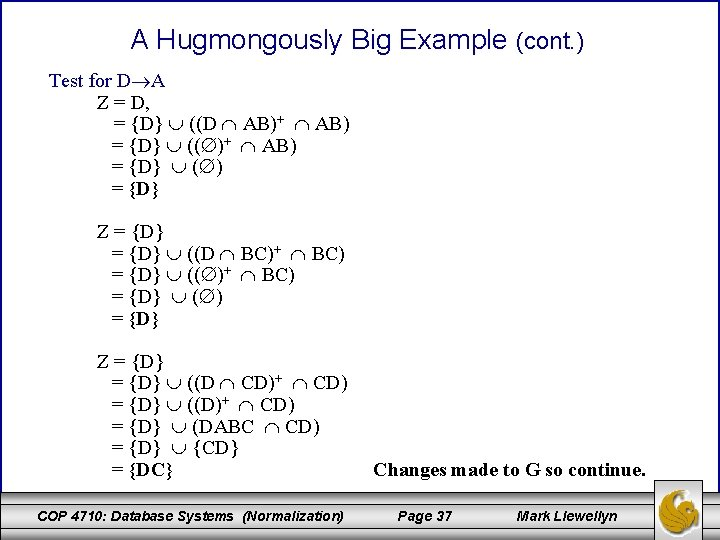A Hugmongously Big Example (cont. ) Test for D A Z = D, = {D} ((D AB)+ AB) = {D} (( )+ AB) = {D} ( ) = {D} Z = {D} ((D BC)+ BC) = {D} (( )+ BC) = {D} ( ) = {D} Z = {D} ((D CD)+ CD) = {D} ((D)+ CD) = {D} (DABC CD) = {D} {CD} = {DC} COP 4710: Database Systems (Normalization) Changes made to G so continue. Page 37 Mark LlewellynA Hugmongously Big Example (cont. ) Test for D A continues on a second pass through D. Z = DC, = {DC} ((DC AB)+ AB) = {DC} (( )+ AB) = {DC} ( ) = {DC} Z = {DC} ((DC BC)+ BC) = {DC} ((C)+ BC) = {D} (CDAB BC) = {D} (BC) = {DBC} Z = {DBC} ((DBC CD)+ CD) = {DBC} ((CD)+ CD) = {DBC} (CDAB CD) = {DBC} {CD} = {DBC} Again changes made to G so continue. COP 4710: Database Systems (Normalization) Page 38 Mark Llewellyn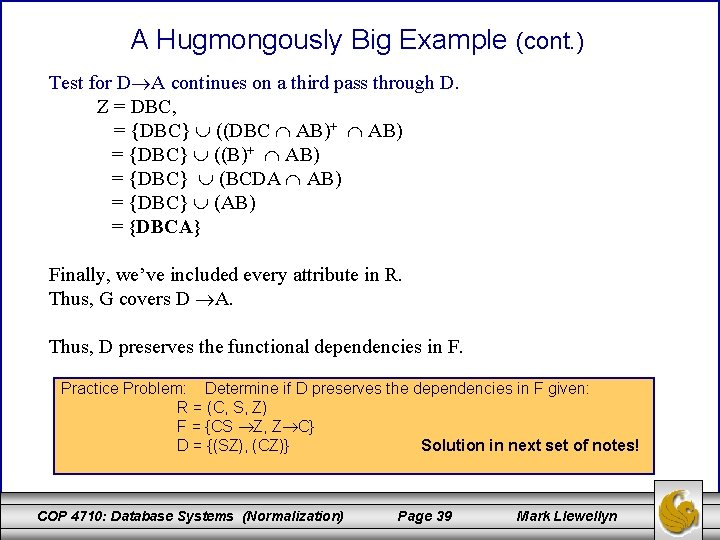A Hugmongously Big Example (cont. ) Test for D A continues on a third pass through D. Z = DBC, = {DBC} ((DBC AB)+ AB) = {DBC} ((B)+ AB) = {DBC} (BCDA AB) = {DBC} (AB) = {DBCA} Finally, we’ve included every attribute in R. Thus, G covers D A. Thus, D preserves the functional dependencies in F. Practice Problem: Determine if D preserves the dependencies in F given: R = (C, S, Z) F = {CS Z, Z C} D = {(SZ), (CZ)} Solution in next set of notes! COP 4710: Database Systems (Normalization) Page 39 Mark Llewellyn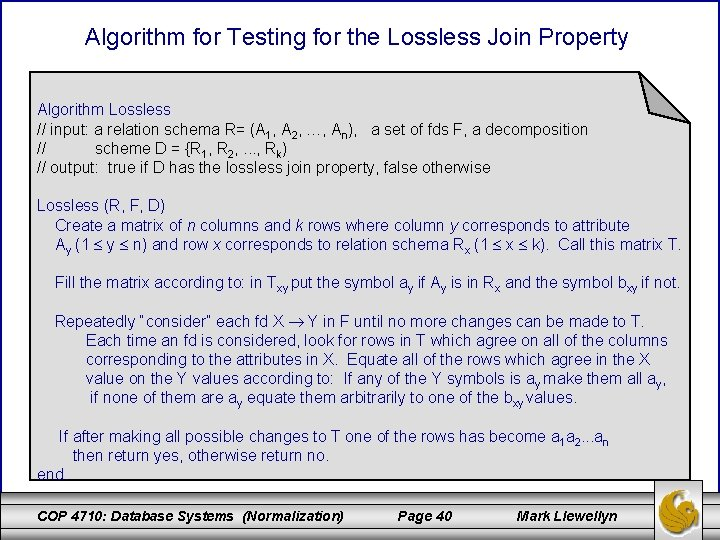Algorithm for Testing for the Lossless Join Property Algorithm Lossless // input: a relation schema R= (A 1, A 2, …, An), a set of fds F, a decomposition // scheme D = {R 1, R 2, . . . , Rk) // output: true if D has the lossless join property, false otherwise Lossless (R, F, D) Create a matrix of n columns and k rows where column y corresponds to attribute Ay (1 y n) and row x corresponds to relation schema Rx (1 x k). Call this matrix T. Fill the matrix according to: in Txy put the symbol ay if Ay is in Rx and the symbol bxy if not. Repeatedly “consider” each fd X Y in F until no more changes can be made to T. Each time an fd is considered, look for rows in T which agree on all of the columns corresponding to the attributes in X. Equate all of the rows which agree in the X value on the Y values according to: If any of the Y symbols is a y make them all ay, if none of them are ay equate them arbitrarily to one of the bxy values. If after making all possible changes to T one of the rows has become a 1 a 2. . . an then return yes, otherwise return no. end. COP 4710: Database Systems (Normalization) Page 40 Mark LlewellynTesting for a Lossless Join - Example Let R = (A, B, C, D, E) F = {A C, B C, C D, DE C, CE A} D = {(AD), (AB), (BE), (CDE), (AE)} initial matrix T: A B C D E (AD) a 1 b 12 b 13 a 4 b 15 (AB) a 1 a 2 b 23 b 24 b 25 (BE) b 31 a 2 b 33 b 34 a 5 (CDE) b 41 b 42 a 3 a 4 a 5 (AE) a 1 b 52 b 53 b 54 a 5 COP 4710: Database Systems (Normalization) Page 41 Mark LlewellynTesting for a Lossless Join – Example (cont. ) Consider each fd in F repeatedly until no changes are made to the matrix: A C: equates b 13, b 23, b 53. . Arbitrarily we’ll set them all to b 13 as shown. A B C D E (AD) a 1 b 12 b 13 a 4 b 15 (AB) a 1 a 2 b 13 b 24 b 25 (BE) b 31 a 2 b 33 b 34 a 5 (CDE) b 41 b 42 a 3 a 4 a 5 (AE) a 1 b 52 b 13 b 54 a 5 COP 4710: Database Systems (Normalization) Page 42 Mark LlewellynTesting for a Lossless Join – Example (cont. ) Consider each fd in F repeatedly until no changes are made to the matrix: B C: equates b 13, b 33. . We’ll set them all to b 13 as shown. A B C D E (AD) a 1 b 12 b 13 a 4 b 15 (AB) a 1 a 2 b 13 b 24 b 25 (BE) b 31 a 2 b 13 b 34 a 5 (CDE) b 41 b 42 a 3 a 4 a 5 (AE) a 1 b 52 b 13 b 54 a 5 COP 4710: Database Systems (Normalization) Page 43 Mark LlewellynTesting for a Lossless Join – Example (cont. ) Consider each fd in F repeatedly until no changes are made to the matrix: C D: equates a 4, b 24, b 34, b 54. . We set them all to a 4 as shown. A B C D E (AD) a 1 b 12 b 13 a 4 b 15 (AB) a 1 a 2 b 13 a 4 b 25 (BE) b 31 a 2 b 13 a 4 a 5 (CDE) b 41 b 42 a 3 a 4 a 5 (AE) a 1 b 52 b 13 a 4 a 5 COP 4710: Database Systems (Normalization) Page 44 Mark LlewellynTesting for a Lossless Join – Example (cont. ) Consider each fd in F repeatedly until no changes are made to the matrix: DE C: equates a 3, b 13. . We set them both to a 3 as shown. A B C D E (AD) a 1 b 12 b 13 a 4 b 15 (AB) a 1 a 2 b 13 a 4 b 25 (BE) b 31 a 2 a 3 a 4 a 5 (CDE) b 41 b 42 a 3 a 4 a 5 (AE) a 1 b 52 a 3 a 4 a 5 COP 4710: Database Systems (Normalization) Page 45 Mark Llewellyn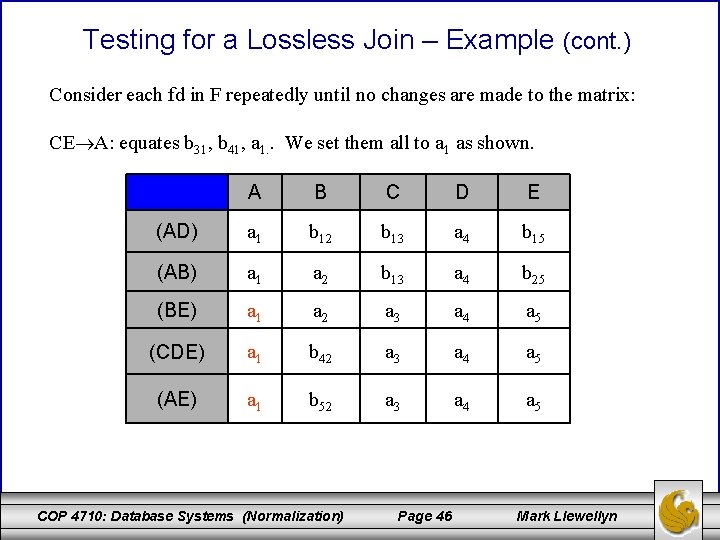Testing for a Lossless Join – Example (cont. ) Consider each fd in F repeatedly until no changes are made to the matrix: CE A: equates b 31, b 41, a 1. . We set them all to a 1 as shown. A B C D E (AD) a 1 b 12 b 13 a 4 b 15 (AB) a 1 a 2 b 13 a 4 b 25 (BE) a 1 a 2 a 3 a 4 a 5 (CDE) a 1 b 42 a 3 a 4 a 5 (AE) a 1 b 52 a 3 a 4 a 5 COP 4710: Database Systems (Normalization) Page 46 Mark Llewellyn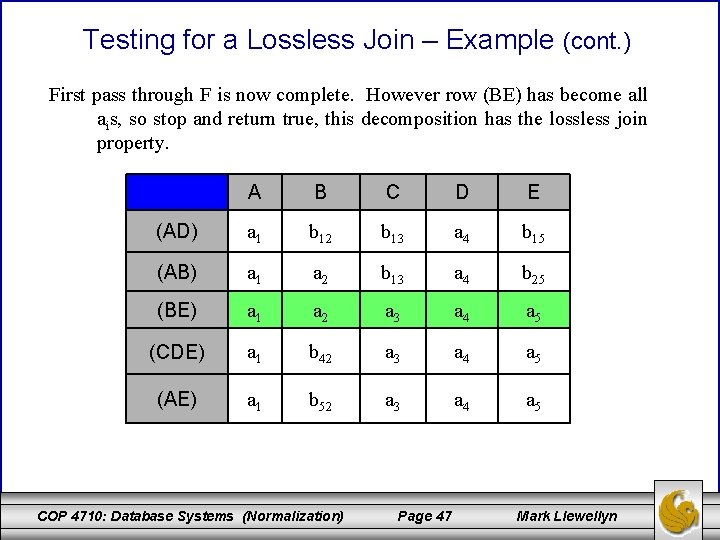Testing for a Lossless Join – Example (cont. ) First pass through F is now complete. However row (BE) has become all ais, so stop and return true, this decomposition has the lossless join property. A B C D E (AD) a 1 b 12 b 13 a 4 b 15 (AB) a 1 a 2 b 13 a 4 b 25 (BE) a 1 a 2 a 3 a 4 a 5 (CDE) a 1 b 42 a 3 a 4 a 5 (AE) a 1 b 52 a 3 a 4 a 5 COP 4710: Database Systems (Normalization) Page 47 Mark Llewellyn